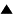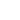# Genetic Algorithms

A genetic algorithm (GA) [1-3] is a computer model of an evolution of a population of artificial individuals. Each individual is characterized by its chromosome Sk, which determines the individual fitness f(Sk); k = 1,..., n; n is a population size. The chromosome is a string of symbols, Sk = (Sk1, Sk2,...,SkN), N is a string length. The symbols Sk1 are interpreted as genes of chromosome Sk .

The evolution process consists of successive generations. At each generation, individuals with high fitness are selected, the chromosomes of selected individuals are recombined and subjected to small mutations. Formally, the scheme of GA can be represented as follows (The population at t-th generation is designated by {Sk(t)}):

• Step 0. Create a random initial population {Sk(0)}.
• Step 1. Evaluate the fitness f(Sk) of each individual Sk in the population {Sk(t)}.
• Step 2. Selecting the individuals Sk according to their fitness f(Sk) and applying genetic operators (recombinations and point mutations) to selected chromosomes, generate the offspring population {Sk(t+1)}.
• Step 3. Repeat the steps 1,2 for t = 0, 1, 2, ... , until some convergence criteria (the maximum fitness in the population ceases to increase, t reaches the certain value) is satisfied.

There are a number of particular GA schemes, which differ in methods of selection, recombination, chromosome representation, etc.

A traditional GA works on the binary string (symbols Ski take the values 0 or1) of fixed length (N = const) and applies fitness-proportionate selection, one-point crossovers, and one-flip mutations.

The fitness-proportionate selection means that during the Step 2 the parents Sk of individuals of the new population are selected with probabilities, which are proportional to their fitness f(Sk). Another example of a selection is the ranking one: a certain number of best the individuals of the population {Sk(t)}are used as parents of a new generation.

The one-point crossover is organized analogously to the biological one: if we have two parents S1 = (S11, S12,...,S1N) and S2 = (S21, S22,..., S2N), their children are (S11,..., S1m, S2,m+1,...,S2N) and (S21,..., S2m, S1,m+1,...,S1N); that is a head and a tail of an offspring chromosome are taken from different parents. Two-point and several point crossovers can be organized in similar manner. Such crossovers are sometimes supplemented by inversions (which are reverses of the symbol order in a part of a chromosome) in order to find the essential combinations of symbols in the strings. Some GAs use a uniform recombination, that is, the symbols of the chromosome of the first offspring are taken from either of the parents (S1 or S2) randomly for any symbol position, whereas the second offspring has remainder symbols; e.g., two children of S1 and S2 can have the following chromosomes: (S11, S22, S13, S14,...,S2N) and (S21, S12, S23, S24,...,S1N).

As optimization technique, the GAs possess an implicit parallelism: different partial effective gene combinations (they are called “schemata”) are searched in parallel manner, simultaneously for all searched combinations. Note, that the smaller is a combination, the quicker it can be found.

The GA scheme is very similar to that of quasispecies. The main difference is that recombinations do not included in the quasispecies model, whereas namely recombinations play the main role to find the new good solutions in GAs (mutation intensity is usually very small in GAs).

Conclusion. GAs are computer program models, which are based on a general understanding of the genetic evolution. GAs are rather universal heuristic optimization methods, which require little knowledge about a problem to be solved. They are also effective methods of combinatorial optimization.

References:

1. Holland, J.H. (1975) Adaptation in Natural and Artificial Systems, Ann Arbor, MI: The University of Michigan Press. 2nd edn. (1992) Boston, MA: MIT Press.

2. Goldberg, D.E. (1989) Genetic Algorithms in Search, Optimization, and Machine Learning, Addison-Wesley.

3. Mitchell, M. (1996) An Introduction to Genetic Algorithms. MIT Press, Cambridge, MA.

 HomeMetasystem Transition TheoryEvolutionary TheoryMathematical Modeling of EvolutionApplied Evolutionary Modeling Up Prev.Next Down